Courses

# Feedback Circuit Electrical Engineering (EE) Notes | EduRev

## Electronic Devices

Created by: Cstoppers Instructors

## Electrical Engineering (EE) : Feedback Circuit Electrical Engineering (EE) Notes | EduRev

The document Feedback Circuit Electrical Engineering (EE) Notes | EduRev is a part of the Electrical Engineering (EE) Course Electronic Devices.
All you need of Electrical Engineering (EE) at this link: Electrical Engineering (EE)

Feedback Circuit

The function of the feedback circuit is to return a fraction of the output voltage to the input of the amplifier. Fig. 1.6 shows the feedback circuit of negative voltage feedback amplifier. It is essentially a potential divider consisting of resistances R1 and R2. The output voltage of the amplifier is fed to this potential divider which gives the feedback voltage to the input. Referring to Fig. 1.6, it is clear that :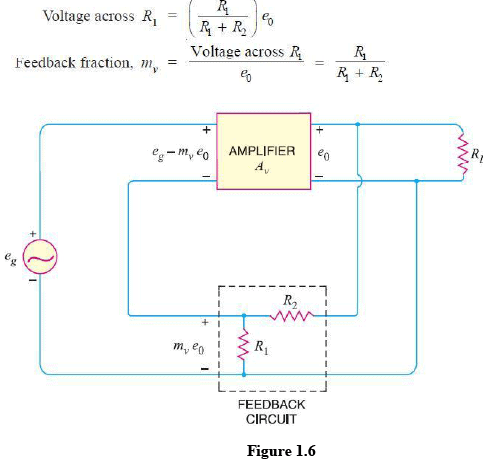Principles of Negative Current Feedback

In this method, a fraction of output current is fedback to the input of the amplifier. In ot her words, the feedback current (If) is proportional to the output current ( Iout) of the amplifier. Fig. 1.7 shows the principles of negative current feedback. This circuit is called current- shunt feedback circuit. A feedback resistor Rf is connected between input and output of the amplifier. This amplifier has a current gain of Ai without feedback. It means that a current I1 at the input terminals of the amplifier will appear as Ai Iin the output circuit i.e., Iout = Ai I1.
Now a fraction mof this output current is fedback to the input through Rf.
The fact that arrowhead shows the feed current being fed forward is because it is negative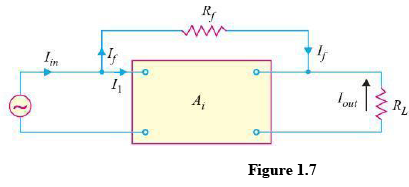Feedback current, If = mi Iout

Note that negative current feedback reduces the input current to the amplifier and hence its current gain.

current Gain with Negative Current Feedback

Referring to Fig. 1.6, we have, Iin = I1 + If = I1 + mi Iout

But Iout = AI1, where Ai is the current gain of the amplifier without feedback. Iin = I1+ mi Ai I1 (ä Iout = Ai I1) Current gain with negative current feedback is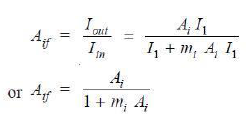This equation looks very much like that for the voltage gain of negative voltage feedback amplifier. The only difference is that we are dealing with current gain rather than the voltage gain.

The following points may be noted carefully :

i) The current gain of the amplifier without feedback is Ai. However, when negative current feedback is applied, the current gain is reduced by a factor (1 + mi Ai).

(ii) The feedback fraction (or current attenuation) mi has a value between 0 and 1.

(iii) The negative current feedback does not affect the voltage gain of the amplifier.

Effects of Negative Current Feedback

The negative current feedback has the following effects on the performance of amplifiers :

(i) Decreases the input impedance. The negative current feedback decreases the input impedance of most amplifiers.

Let
Zin = Input impedance of the amplifier without feedback
Z ′in = Input impedance of the amplifier with negative current feedback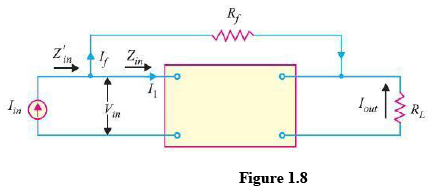Referring to Fig. 1.8, we have,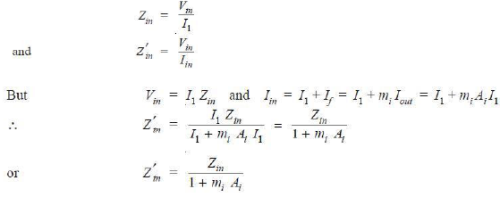Thus the input impedance of the amplifier is decreased by the factor (1 + mi Ai). Note the primary  difference between negative current feedback and negative voltage feedback. Negative current feedback decreases the input impedance  of the amplifier while negative voltage feedback  increases the input impedance of the amplifier.

Increases the output impedance. It can be proved that with negative current feedback, the output impedance of the amplifier is increased by a factor (1 + mi Ai).

Z ′out = Zout (1 + mi Ai)

where

Zout = output impedance of the amplifier without feedback
Z ′out = output impedance of the amplifier with negative current feedback
The reader may recall that with negative voltage feedback, the output impedance of the amplifier is decreased.

Increases bandwidth. It can be shown that with negative current feedback, the bandwidth of the amplifier is increased by the factor (1 + mi Ai).
BW ′ = BW (1 + mi Ai)

where
BW = Bandwidth of the amplifier without feedback
BW ′ = Bandwidth of the amplifier with negative current feedback

The Feedback Voltage Amplifier (Series- Shunt)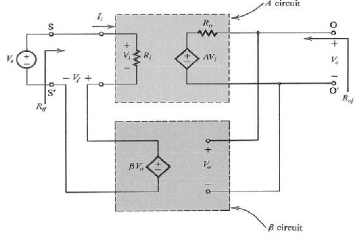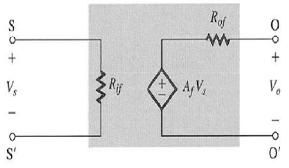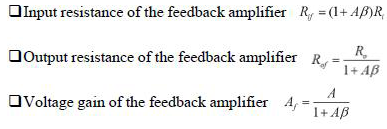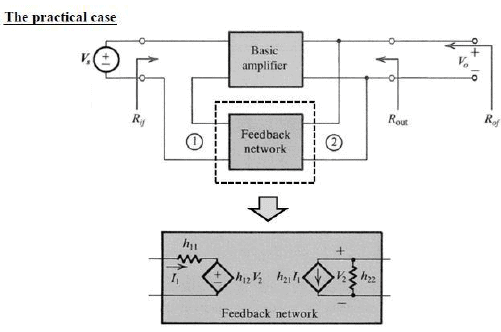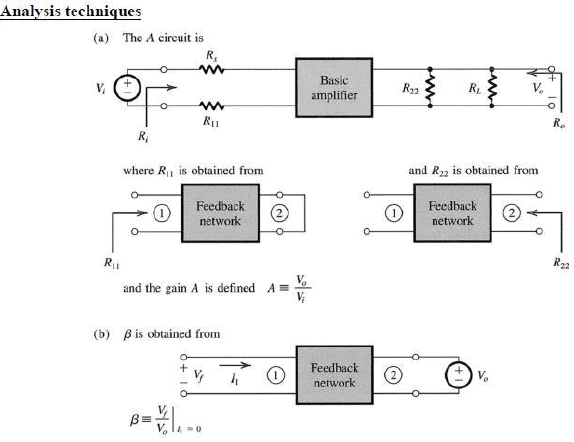Offer running on EduRev: Apply code STAYHOME200 to get INR 200 off on our premium plan EduRev Infinity!

,

,

,

,

,

,

,

,

,

,

,

,

,

,

,

,

,

,

,

,

,

;